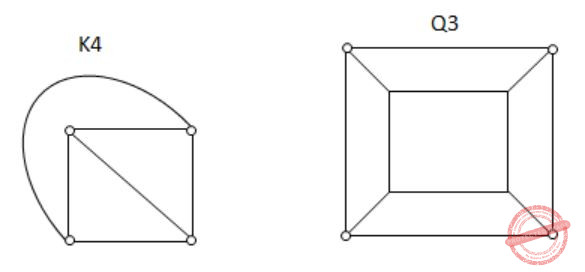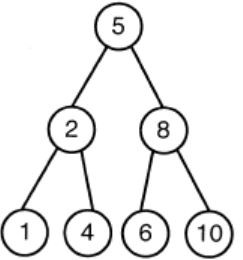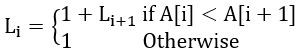## GATE 2011

 Question 1

The simplified SOP (sum of product) form of the boolean expressionABCDQuestion 1 Explanation:Question 2

The minimum number of D flip-flops needed to design a mod-258 counter is

 A 9 B 8 C 512 D 258
Question 2 Explanation:
Let n is the number of flip-flops.
The max Mod values is 2n.
So 2n ≥ 258 ⇒ n = 9
 Question 3

Which one of the following circuits is NOT equivalent to a 2-input XNOR (exclusive NOR) gate?

 ABCDQuestion 3 Explanation:
All options except option ‘D’ gives EX-NOR gates.
 Question 4

A thread is usually defined as a "light weight process" because an operating system (OS) maintains smaller data structures for a thread than for a process. In relation to this, which of the following is TRUE?

 A On per-thread basis, the OS maintains only CPU register state B The OS does not maintain a separate stack for each thread C On per-thread basis, the OS does not maintain virtual memory state D On per thread basis, the OS maintains only scheduling and accounting information
Question 4 Explanation:
A) False, because on per thread basis OS maintains register, stack and program counter.
B) False, OS do maintain a separate stack for each thread.
C) True
D) False
 Question 5

K4 and Q3 are graphs with the following structures.Which one of the following statement is TRUE  in relation to these graphs?

 A K4 is planar while Q3 is not B Both K4 and Q3 are planar C Q3 is planar while K4 is not D Neither K4 not Q3 is planar
Question 5 Explanation:Both the above graphs are planar.
 Question 6

If the difference between the expectation of the square of a random variable (E[X2]) and the square of the expectation of the random variable (E[X])2 is denoted by R, then

 A R = 0 B R < 0 C R ≥ 0 D R > 0
Question 6 Explanation:
We know that difference of E(X2) and E(X))2 is nothing but variance or V(X) which is always greater than or equal to zero.
So the answer will be R≥0.
 Question 7

The lexical analysis for a modern computer language such as Java needs the power of which one of the following machine models in a necessary and sufficient sense?

 A Finite state automata B Deterministic pushdown automata C Non-Deterministic pushdown automata D Turing machine
Question 7 Explanation:
Lexical Analysis is implemented by finite automata.
 Question 8

Let the page fault service time to 10 ms in a computer with average memory access time being 20 ns. If one page fault is generated for every 106 memory accesses, what is the effective access time for the memory?

 A 21 ns B 30 ns C 23 ns D 35 ns
Question 8 Explanation:
P = page fault rate
EA = p × page fault service time + (1 – p) × Memory access time
= 1/106×10×106+(1-1/106)×20 ≅ 29.9 ns
 Question 9

Consider a hypothetical processor with an instruction of type LW R1, 20 (R2), which during execution reads a 32-bit word from memory and stores it in a 32-bit register R1. The effective address of the memory location is obtained by the addition of constant 20 and the contents of register R2. Which of the following best reflects the addressing mode implemented by this instruction for the operand memory?

Question 9 Explanation:
Here 20 will act as base and content of R2 will be index.
 Question 10

What does the following fragment of C-program print?

```char c[] = "GATE2011";
char *p =c;
printf("%s", p + p - p) ; ```
 A GATE2011 B E2011 C 2011 D 011
Question 10 Explanation:
p is the starting address of array.
p - p = 4, and p+4 will be pointing to the fifth position in the array 'c'. So printf starts printing from 2 and prints 2011.
 Question 11

A max-heap is a heap where the value of each parent is greater than or equal to the value of its children. Which of the following is a max-heap?

 ABCDQuestion 11 Explanation:
Heap is a complete binary tree
Option A: It violates the property of complete binary tree.
Option C: 8 is greater than 5. The root value is always greater than his children. So, the above tree is violating the property of max heap.
Option D: 10 is greater than 8. The root value is always greater than his children. So, the above tree is violating the property of max heap.
 Question 12

An algorithm to find the length of the longest monotonically increasing sequence of numbers in an array A[0:n-1] is given below. Let Li denote the length of the longest monotonically increasing sequence starting at index i in the array

```Initialize Ln-1=1.
For all i such that 0≤i≤n-2```

Finally the length of the longest monotonically increasing sequence is Max(L0, L1, ..., Ln-1).

Which of the following statements is TRUE?

 A The algorithm uses dynamic programming paradigm B The algorithm has a linear complexity and uses branch and bound paradigm C The algorithm has a non-linear polynomial complexity and uses branch and bound paradigm D The algorithm uses divide and conquer paradigm
Question 12 Explanation:
Key Note of Dynamic programming: 1. Dynamic programming is when you use past knowledge to make solving a future problem easier.
2. Dynamic programming is a technique used to avoid computing multiple time the same sub-problem in a recursive algorithm.
Note: It is neither backtracking nor branch and bound because we are not branching anywhere in the solution space. The algorithm is not divide and conquer as we are not dividing the problem and then merging the solution
 Question 13

Let P be a regular language and Q be a context-free language such that Q ⊆ P. (For example, let P be the language represented by the regular expression p*q* and Q be [pnqn | n ∈ N]). Then which of the following is ALWAYS regular?

 A P ∩ Q B P – Q C Σ* – P D Σ* – Q
Question 13 Explanation:
Exp: Σ* - P is the complement of P so it is always regular,
since regular languages are closed under complementation.
 Question 14

In a compiler, keywords of a language are recognized during

 A parsing of the program B the code generation C the lexical analysis of the program D dataflow analysis
Question 14 Explanation:
Any identifier is also a token so it is recognized in lexical Analysis.
 Question 15

A layer-4 firewall (a device that can look at all protocol headers up to the transport layer) CANNOT

 A block entire HTTP traffic during 9:00PM and 5:00AM B block all ICMP traffic C stop incoming traffic from a specific IP address but allow outgoing traffic to the same IP address D block TCP traffic from a specific user on a multi-user system during 9:00PM and 5:00AM
Question 15 Explanation:
(A) It is possible to block entire HTTP traffic by blocking port no.80.
(B) Possible because it is network layer protocol.
(C) Possible because SP address is present in Network layer.
(D) Not possible, because to block specific user, we need user id which is present in Application layer.
 Question 16

If two fair coins are flipped and at least one of the outcomes is known to be a head, what is the probability that both outcomes are heads?

 A 1/3 B 1/4 C 1/2 D 2/3
Question 16 Explanation:
Sample space = {HH, HT, TH}
Required probability = 1/3
There are 16 questions to complete.

Register Now